# Shape operator on a hypersurface

(Redirected from Shape operator)

## Definition

### For a hypersurface in any dimension

Suppose$M$ is a$n$-dimensional manifold embedded inside$\R^{n+1}$. The shape operator on$M$ associates, to every point$p \in M$, a linear map from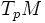$T_pM$ to$T_pM$, given by: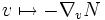$v \mapsto - \nabla_vN$

where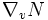$\nabla_vN$ is the component of$D_vN$ (the covariant derivative of the normal in terms of$v$) in the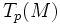$T_p(M)$-direction.

Equivalently, the shape operator is the differential of the Gauss map for the hypersurface$M$, namely the map: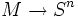$M \to S^n$

that sends a point in$M$ to the normal direction at that point.

The shape operator can be viewed as a section of the bundle$\Gamma(T^*M) \otimes \Gamma(TM)$.

### For a regular surface in 3-space

This is the special case of the above, in the situation where$M$ is a regular surface inside$\R^3$.